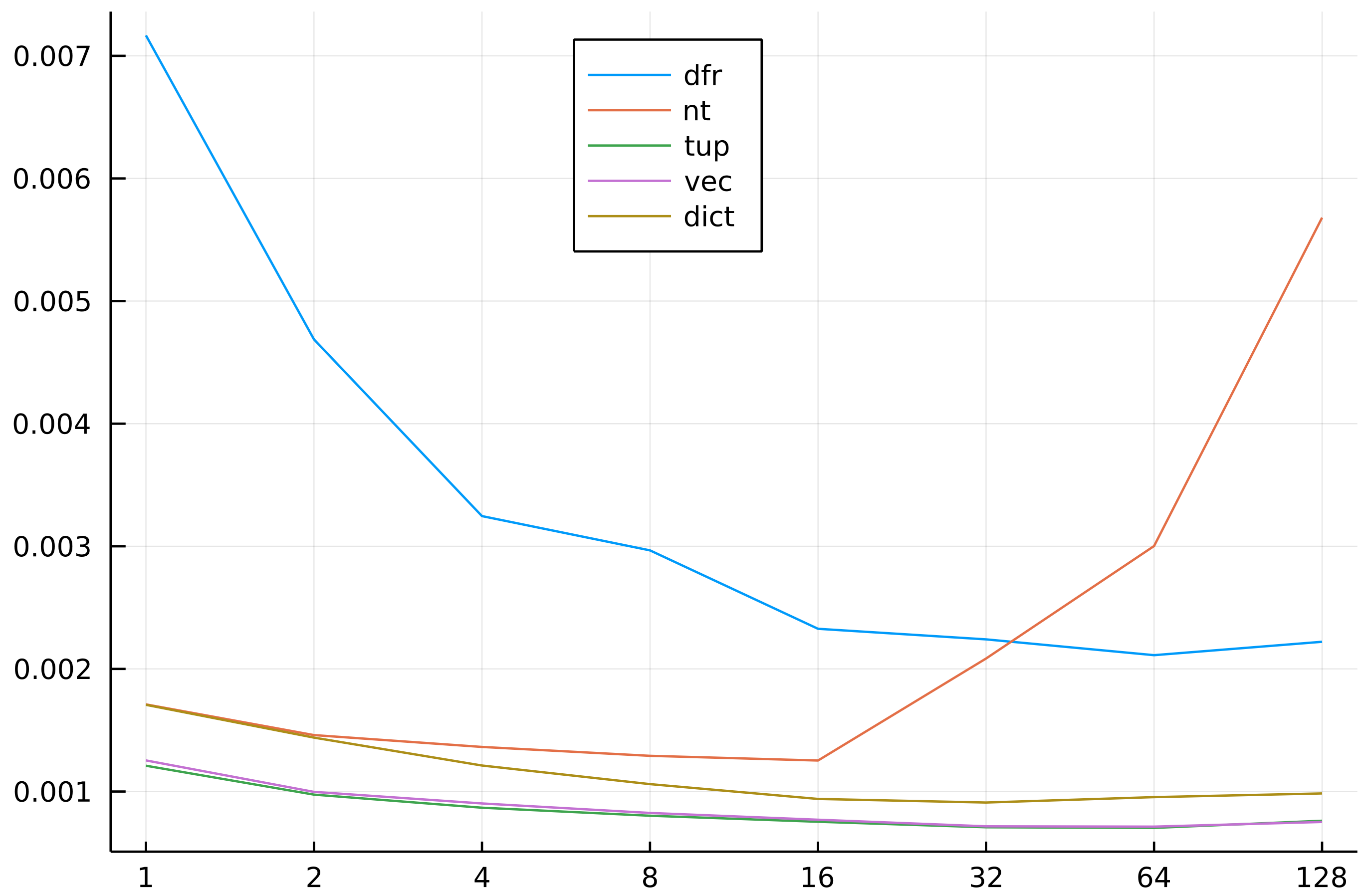# Introduction

I really like the fact that push! is a convenient and fast way to add rows to a DataFrame in DataFrames.jl. Recently I have noticed that users also employ it quite often. Therefore I thought to post some benchmarks of timing of push! operation for different types of rows pushed.

The tests were run under Julia 1.6.1, DataFrames.jl 1.1.1, and BenchmarkTools.jl 1.0.0.

# The benchmarks

In the benchmark I thought of testing one of the most common scenarios:

• pushed columns have a homogeneous type;
• we use the default settings of keyword arguments: cols=:setequal and promote=false.

In the test we check the time to push 10,000 rows to a data frame and consider the following supported types of rows pushed:

• DataFrameRow,
• NamedTuple,
• Tuple,
• Vector,
• Dict.

I want to see which option is the fastest and how does the push! speed depend on the number of columns pushed.

Here is the test code:

julia> using BenchmarkTools

julia> using DataFrames

julia> function test_push(v)
df = DataFrame(Symbol.("x", 1:length(v)) .=> Ref(Int[]))
foreach(r -> push!(df, r), v)
return df
end
test_push (generic function with 1 method)

julia> function run_test(n)
rows_dfr = [DataFrame(Symbol.("x", 1:n) .=> i)[1, :] for i in 1:10000];
rows_nt = NamedTuple.(rows_dfr)
rows_tup = Tuple.(rows_dfr)
rows_vec = Vector.(rows_dfr)
rows_dict = Dict.(pairs.(rows_dfr))
return (n=n,
dfr=@belapsed(test_push($rows_dfr)), nt=@belapsed(test_push($rows_nt)),
tup=@belapsed(test_push($rows_tup)), vec=@belapsed(test_push($rows_vec)),
dict=@belapsed(test_push(\$rows_dict)))
end
run_test (generic function with 1 method)

julia> @time res = run_test.(2 .^ (0:7)) |> DataFrame;
491.489886 seconds (10.05 G allocations: 318.857 GiB, 15.44% gc time, 1.90% compilation time)


As you can see the test is quite time consuming (and that is why the results are worth posting).

Before I move forward let me comment on one line in the code, namely:

df = DataFrame(Symbol.("x", 1:length(v)) .=> Ref(Int[]))


Many Julia users will immediately spot that the Symbol.("x", 1:length(v)) .=> Ref(Int[]) pattern will lead to Int[] vector being reused in all Pairs in the produced vector, e.g.

julia> tmp = Symbol.("x", 1:2) .=> Ref(Int[])
2-element Vector{Pair{Symbol, Vector{Int64}}}:
:x1 => []
:x2 => []

julia> tmp.second === tmp.second
true


Normally creation of such collections is strongly discouraged as it can lead to hard-to-catch bugs. Instead a typical recommendation is to use comprehension which guarantees that Int[] vector is freshly allocated in each iteration, e.g.:

julia> tmp = [Symbol("x", i) => Int[] for i in 1:2]
2-element Vector{Pair{Symbol, Vector{Int64}}}:
:x1 => []
:x2 => []

julia> tmp.second === tmp.second
false


So why it was OK to use it in my code? The reason is that DataFrame constructor copies all columns by default so aliased columns do not get stored in the produced data frame object.

Going back to our main thread, here are the collected timings:

julia> res
8×6 DataFrame
Row │ n      dfr         nt          tup         vec         dict
│ Int64  Float64     Float64     Float64     Float64     Float64
─────┼───────────────────────────────────────────────────────────────────
1 │     1  0.00716675  0.00171069  0.00121046  0.00125374  0.00170707
2 │     2  0.0093752   0.00292054  0.00194945  0.0019941   0.00287937
3 │     4  0.0129853   0.00545446  0.00347197  0.0036109   0.00484835
4 │     8  0.0237338   0.0103292   0.0064206   0.0066019   0.00848298
5 │    16  0.0372345   0.0200449   0.0120538   0.0123195   0.0150318
6 │    32  0.0717086   0.0667088   0.0226685   0.0229196   0.0291269
7 │    64  0.13517     0.192137    0.045016    0.0456765   0.0610755
8 │   128  0.284317    0.727081    0.0974844   0.0962173   0.126006


To simplify assessment of the results let me plot them. In order to improve the readability of the comparisons below I plot the time per one column (which means that if the push! operation scales well with number of columns the plots should be slightly decreasing). Here is the code producing the plot:

julia> using Plots

julia> plot(Matrix(res)[:, 2:end] ./ res.n,
labels=permutedims(names(res)[2:end]),
xticks=(axes(res, 1), res.n), legend=:top)


And the plot itself:# Conclusions

Here are the take-aways:

• pushing Tuple or Vector is fastest; the reason is that in this case we do not care about column names;
• Dict is quite fast; the overhead is mostly due to column name lookup;
• DataFrameRow is slow; this is not surprising, as it is type unstable;
• NamedTuple is comparable to Dict for small number of columns but it does not scale well and for large number of columns it is very slow.

A simple take-away is: if you generate a lot of data (e.g. in a simulation) then prefer to produce a Vector or a Tuple for push!ing into a DataFrame as this is fastest.